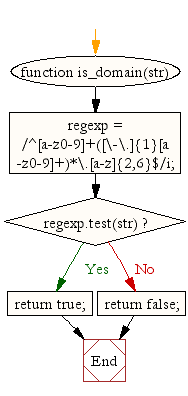# JavaScript: Check whether a given value represents a domain or not

## JavaScript validation with regular expression: Exercise-18 with Solution

Write a JavaScript function to check whether a given value represents a domain.

Sample Solution:-

HTML Code:

``````<!DOCTYPE html>
<html>
<meta charset="utf-8">
<title>Write a JavaScript function to check whether a given value represents a domain or not</title>
<body>

</body>
</html>
```
```

JavaScript Code:

``````function is_domain(str)
{
regexp = /^[a-z0-9]+([\-\.]{1}[a-z0-9]+)*\.[a-z]{2,6}\$/i;

if (regexp.test(str))
{
return true;
}
else
{
return false;
}
}

console.log(is_domain('www.example.com'));

console.log(is_domain('www.npm.co.uk'));

console.log(is_domain('http://www.example.com'));

console.log(is_domain('https://www.example.com'));

console.log(is_domain('www.example.com'));
```
```

Sample Output:

```true
true
false
false
true
```

Flowchart:Live Demo:

See the Pen javascript-regexp-exercise-18 by w3resource (@w3resource) on CodePen.

Improve this sample solution and post your code through Disqus

What is the difficulty level of this exercise?

Test your Programming skills with w3resource's quiz.

﻿